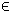## 26LCP Problem

The general formulation in TOMLAB for a linear complementarity problem is:
 min x
f(x) = cT x

s/t
 xL ≤ x ≤ xU bL ≤ A x ≤ bU
(30)
where c, x, xL, xURn, ARm1 × n, and bL,bURm1. Equality constraints are defined by setting the lower bound to the upper bound.

The complementarity conditions can be any combination of decision variables and linear constraints:
• x(i) ⊥ x(j)
• x(i) ⊥ A(j,:) x
• A(i,:) xA(j,:) x
Example problem:

The following file defines a problem in TOMLAB.

File: tomlab/quickguide/lcpQG.m

Open the file for viewing, and execute lcpQG in Matlab.
```% lcpQG is a small linear complementary quick guide example
%
% minimize f: 2*x(1) + 2*x(2) - 3*x(3) - 3*x(4) - 60;
%
% Variable bounds:
%    x(1:2) >= 0, <= 50;
%    x(3:4) unbounded
%    x(5:10) >= 0;
%
% subject to:
%
%    c1: x(1) + x(2) + x(3) - 2*x(4) - 40 <= 0;
%
%    F1: 0 = 2*x(3) - 2*x(1) + 40 - (x(5) - x(6) - 2*x(9));
%    F2: 0 = 2*x(4) - 2*x(2) + 40 - (x(7) - x(8) - 2*x(10));
%
%    g1: 0 <= x(3) + 10            complements  x(5) >= 0;
%    g2: 0 <= -x(3) + 20           complements  x(6) >= 0;
%    g3: 0 <= x(4) + 10            complements  x(7) >= 0;
%    g4: 0 <= -x(4) + 20           complements  x(8) >= 0;
%    g5: 0 <= x(1) - 2*x(3) - 10   complements  x(9) >= 0;
%    g6: 0 <= x(2) - 2*x(4) - 10   complements  x(10) >= 0;
%
% These constraints above are written as 0 <= c(x) but we have to
% move the constant terms out of the linear expressions:
%
%    c1: x(1) + x(2) + x(3) - 2*x(4) <= 40
%
%    F1: -40 =  2*x(3) - 2*x(1) - x(5) + x(6) + 2*x(9);
%    F2: -40 =  2*x(4) - 2*x(2) - x(7) + x(8) + 2*x(10);
%
%    g1: -10 <= x(3)             complements  x(5) >= 0;
%    g2: -20 <= -x(3)            complements  x(6) >= 0;
%    g3: -10 <= x(4)             complements  x(7) >= 0;
%    g4: -20 <= -x(4)            complements  x(8) >= 0;
%    g5:  10 <= x(1) - 2*x(3)    complements  x(9) >= 0;
%    g6:  10 <= x(2) - 2*x(4)    complements  x(10) >= 0;
%
% An MPEC from F. Facchinei, H. Jiang and L. Qi, A smoothing method for
% mathematical programs with equilibrium constraints, Universita di Roma
% Technical report, 03.96. Problem number 7

Name = 'bilevel1';

% Number of variables:   10
% Number of constraints: 9

x_L = zeros(10,1);
x_L(3:4) = -inf;

x_U = inf*ones(size(x_L));
x_U(1:2) = 50;

c = [2 2 -3 -3 0 0 0 0 0 0 ]';

mpec = sparse( [
5     0     4     0     0     0
6     0     5     0     0     0
7     0     6     0     0     0
8     0     7     0     0     0
9     0     8     0     0     0
10    0     9     0     0     0]);

b_L = [-inf, -40, -40, -10, -20, -10, -20, 10, 10 ]';
b_U = [ 40, -40, -40, inf, inf, inf, inf, inf, inf]';

A =[
1     1     1    -2     0     0     0     0     0     0
-2     0     2     0    -1     1     0     0     2     0
0    -2     0     2     0     0    -1     1     0     2
0     0     1     0     0     0     0     0     0     0
0     0    -1     0     0     0     0     0     0     0
0     0     0     1     0     0     0     0     0     0
0     0     0    -1     0     0     0     0     0     0
1     0    -2     0     0     0     0     0     0     0
0     1     0    -2     0     0     0     0     0     0
];

x_0 = ones(10,1);

Prob = lcpAssign(c, x_L, x_U, x_0, A, b_L, b_U, mpec, Name);

Prob.KNITRO.options.ALG = 3;
Prob.PriLevOpt = 2;
Result = tomRun('knitro',Prob,2);

x = Result.x_k;

% The slack values:
s = x(11:end)
x = x(1:10)

A = Prob.orgProb.A;
Ax = A*x;

% The last 6 elements of Ax, on the original form is:

Ax1 = Ax(4:9) + [10,20,10,10,-10,-10]'

% ... in a scalar product with the corresponding elements of x,
% should be zero:

l = x(5:10)
Ax1'*l```